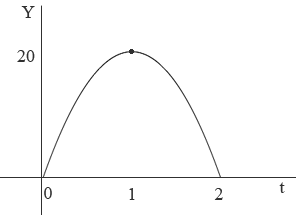SEARCH HOMEMath Central Quandaries & QueriesQuestion from Michael: Football is thrown from a 10-yard line. It reaches its highest height of 20 yards. It lands on the 50-yard line after 2 seconds. What is the equation of the parabola that models this throw? I really need help as I've been on this for the longest amount of time.Hi Michael,

I drew a diagram of height of the football in yards against time in seconds and put on the information you know.This is a parabola so its equation is

$y = a t^2 + bt + c$

for some constants a, b and c. Since a parabola is symmetric around its vertex so the height of 20 yards occurs when t = 1 second. You know that y = 0 when t = 0, y = 0 when t = 2, and y = 20 when t = 1. These facts allow you to determine a, b and c. I expect however that you want a parabola relating x and y not t and y.

If you ignore air friction then the flight of the football in the x direction has zero acceleration so x is a linear function of t. That is for some constants c and k, $x = kt + c.$ You know that x = 20 when t = 0 and x = 50 when t = 2. These fact allow you to determine c and k.

Solve the resulting equation for t and substitute into the equation relating y and t. This gives you a quadratic equation for y in terms of x. Simplify.

Verify that the resulting equation satisfies the data give.

Write back if you need more assistance,
PennyMath Central is supported by the University of Regina and The Pacific Institute for the Mathematical Sciences.